Function Repository Resource:

# SubspaceAngle

Compute the angle between two subspaces

Contributed by: Jan Mangaldan
 ResourceFunction["SubspaceAngle"][u,v] gives the angle between two subspaces specified by the columns of the matrices u and v.

## Details and Options

The matrices u and v must be numerical matrices with the same number of rows.
Vectors are treated as column matrices.
If the elements of u or v are exact numbers, ResourceFunction["SubspaceAngle"] begins by applying N to them.
ResourceFunction["SubspaceAngle"] gives an angle in radians.

## Examples

### Basic Examples (1)

Compute the angle between two subspaces:

 In:=Out=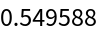### Scope (2)

Find the subspace angle between a vector and a matrix:

 In:=Out=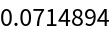Find the subspace angle between different sets of columns of a complex matrix:

 In:=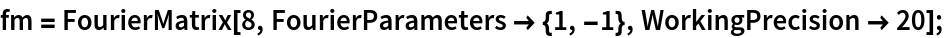In:=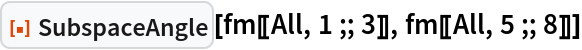Out=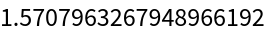### Properties and Relations (2)

For two vectors, SubspaceAngle gives the same result as VectorAngle:

 In:=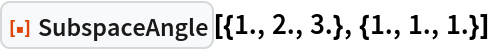Out=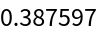In:=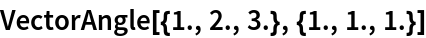Out=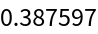Use SubspaceAngle to compute the angle between two planes by specifying the vectors spanning each plane as matrix columns:

 In:=Out=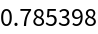Use DihedralAngle to compute the same result:

 In:=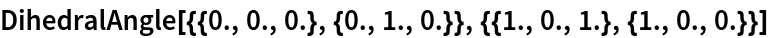Out=## Version History

• 1.0.0 – 08 February 2023